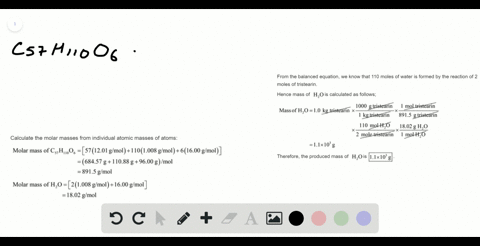Sign up for our free STEM online summer camps starting June 1st!View Summer Courses### The fat stored in a camel's hump is a source of b…

01:43Auburn University Main Campus

Need more help? Fill out this quick form to get professional live tutoring.

Get live tutoring
Problem 100

A chemical plant uses electrical energy to decompose aqueous solutions of NaCl to give $\mathrm{Cl}_{2}, \mathrm{H}_{2},$ and $\mathrm{NaOH} :$
$$2 \mathrm{NaCl}(a q)+2 \mathrm{H}_{2} \mathrm{O}(l) \longrightarrow 2 \mathrm{NaOH}(a q)+\mathrm{H}_{2}(g)+\mathrm{Cl}_{2}(g)$$
If the plant produces $1.5 \times 10^{6} \mathrm{kg}\left(1500$ metric tons) of $\mathrm{Cl}_{2}\right.$ daily, estimate the quantities of $\mathrm{H}_{2}$ and $\mathrm{NaOH}$ produced.

Check the explanation

## Discussion

You must be signed in to discuss.

## Video Transcript

So in this problem, we're given every actually question and in the waves we're given and the mass ofthe chlorine which is being produced here. And we have to find out the mass of sodium hydroxide and hydrogen gas being produced. So what we have to do here first, we will find out the number of malls off chlorine, which is being proposed because in a balanced equation, the only way to compare the mass ofthe one react and our product with the mass off another react under project is to compare their number of malls. So the first thing we have to do, we need to find out the number of malls of chlorine is which is being produced. And from there we can find out the number of most ofthe NCL, which is required, perform that much number of mull of chlorine. And from that number of sodium chloride, we can find out how many number of most ofthe sodium hydroxide and how many number of months off Hydra is and will be produced. And from there you can find out the mass of sodium hydroxide and hydrogen which were reproduced. So our first thing is we'LL find out the number of most ofthe glory in which is being produced. A number of most ofthe growing with the massive grain. Or it's smaller mass to the massive green, which is produces one point five names. And there were six. Busy Our since all the solar masses are ah, tilting Graham. We'LL write it as one point five times ten to the bar. Nine graham into the poor nine Graham over the molar Mass. It's off glory, in which is thirty five point four five grandpa are more so This will give us a value off four point two three times ten to the four seven more So this is the number of mall off chlorine, which is being produced. So from the balanced equation, we can see that from all of sodium chloride can produce one mole of chlorine that men's this much more of sodium. Ah, this possible off chlorine will be produced by these times to matloff sodium chloride, and that means the number of walls of sodium chloride will be two times four point two three grams to know the worst on mall and which is equal to eight point four six times to the words on more. So this is the number of mall off a sodium chloride being produced now. From here, we can find out the number of Melo Story, um, hydroxide and hydrogen gas, which will be produced so we can see that to mono. Sodium chloride can produce two more low sodium hydroxide. That means this much more of sodium chloride will produce the same number of moral sodium hydroxide that men's number of mall off. Sorry. Um hydroxide, which is being produced, is same as eight point four six. Time stood with the bar seven more, and from here we can find the massive sodium hydroxide, which is being produced. So the mass of sodium hydroxide being produced will be simply in the number of most of sodium hydroxide times its molar mass, which is of forty Grandpa Ramon. And from here we will have value off three point three eight times tent with upon nine graham. So this is them massive sodium hydroxide, which is being produced now. We'LL find a mass of hydrogen, which is being produced, so from here against see that to mall of sodium chloride can produce one more of hydrogen. That means this much Matloff sodium chloride will produce. It's half mole off half off the small off hydrogen gas and half of this small is basically this. So the number of matloff hydra zin is the half off. Then number of mole off a sodium chloride, which is four point two three times into the purse on mall. And that means the mass ofthe dead Risen, which is produced, will be four point two three times ten to the first seven mall times the molar mass of hide Risen, which is two point zero six Grandpa Ramel. And from here we'LL get Avello off eight point five three times ten to the bar, seven gram off fight Rosen. So this is the massive hydrogen, which will be produced from here. And this is the mess off sodium hydroxide which will be former produced from this direction.